#### You may also like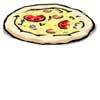### Pizza Portions

My friends and I love pizza. Can you help us share these pizzas equally?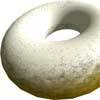### Doughnut

How can you cut a doughnut into 8 equal pieces with only three cuts of a knife?### Pies

Grandma found her pie balanced on the scale with two weights and a quarter of a pie. So how heavy was each pie?

# Fun Time

##### Age 7 to 11Challenge Level

You all realised that you need to work backwards, or from the end of the question, to answer this problem. Thomas, Serge, Runi and Sam from Newberries Primary School all found the correct length of time that Asha and Kishan spent at the fair to be five hours. Sam explains how he worked this out: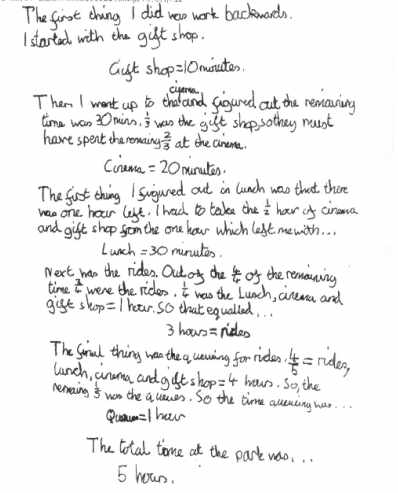Lucy from St John's R. C. Primary School used a pie chart to calculate the answer: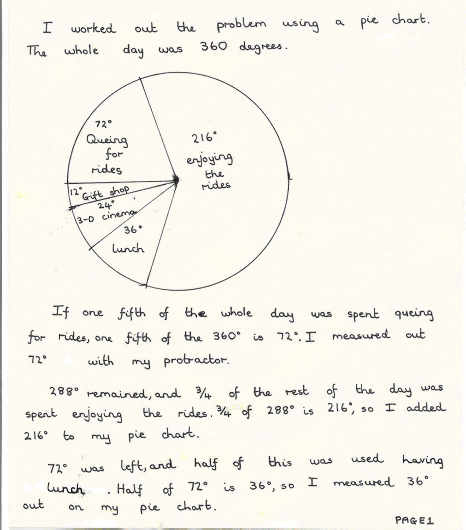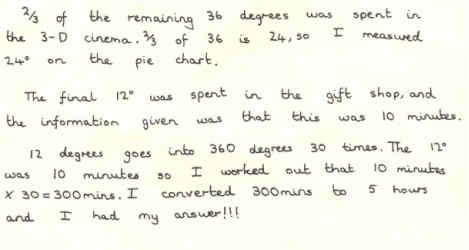Alice and Gwen, who are both from The Downs C.E.P School, represented their solution as a rectangle: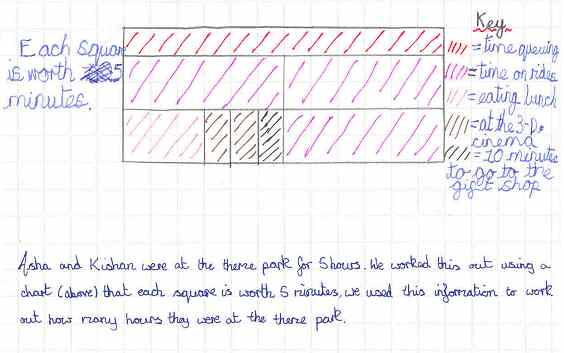Terri also sent us her answer to this question:

After we've counted queuing for the rides, they have $\frac{4}{5}$ of the day left.
After we've counted the rides themselves, they have $\frac{1}{4} \times \frac{4}{5} = \frac{1}{5}$ of the day left.
After we've counted lunch, they have $\frac{1}{2} \times \frac{1}{5} = \frac{1}{10}$ of the day left.
After we've counted the cinema, they have $\frac{1}{3} \times \frac{1}{10} = \frac{1}{30}$ of the day to spend in the gift shop.
But this is 10 minutes. So $\frac{1}{30}$ of the day was $10$ minutes, so the whole time must have been $30 \times 10=300$ minutes, which is $5$ hours.